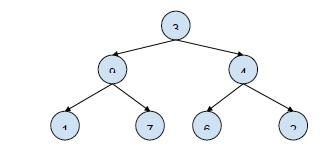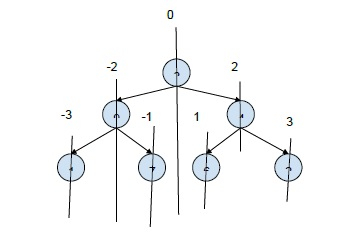# Print all root to leaf paths with there relative positions in C++

In this problem, we are given a binary tree. And we have to print all the paths from the root to the leaf of the tree. Also, add underscore “_” to show the relative positions of the nodes.

Let’s take an example to understand the topic better −

Input −Output −

_ _ 3
_ 9
1
_3
9
_7
3
_ 4
_ _ 2
3
9 4
1 7 6 2
3
_ 4
6

To solve this problem, we will use the concept of the vertical order of the elements of the tree.Based on this, we will print the path from root to leaf.

## Algorithm

Step 1: Traverse the binary tree using preorder traversal. And on traversal calculate the horizontal distance based on the order. The horizontal distance of root is 0 and processed as the above diagram.
Step 2: And on traversing to the leaf node, print the path with an underscore “_” at the end.

## Example

Live Demo

#include<bits/stdc++.h>
using namespace std;
#define MAX_PATH_SIZE 1000
struct Node{
char data;
Node *left, *right;
};
Node * newNode(char data){
struct Node *temp = new Node;
temp->data = data;
temp->left = temp->right = NULL;
return temp;
}
struct PATH{
int horizontalDistance;
char key;
};
void printPath(vector < PATH > path, int size){
int minimumhorizontalDistance = INT_MAX;
PATH p;
for (int it=0; it<size; it++){
p = path[it];
minimumhorizontalDistance = min(minimumhorizontalDistance, p.horizontalDistance);
}
for (int it=0; it < size; it++){
p = path[it];
int noOfUnderScores = abs(p.horizontalDistance -minimumhorizontalDistance);
for (int i = 0; i < noOfUnderScores; i++) cout<<"_ ";
cout<<p.key<<endl;
}
cout<<"\nNext Path\n";
}
void printAllRtLPaths(Node *root, vector < PATH > &AllPath, int horizontalDistance, int order ){
if(root == NULL)
return;
if (root->left == NULL && root->right == NULL){
AllPath[order] = (PATH { horizontalDistance, root->data });
printPath(AllPath, order+1);
return;
}
AllPath[order] = (PATH { horizontalDistance, root->data });
printAllRtLPaths(root->left, AllPath, horizontalDistance-1, order+1);
printAllRtLPaths(root->right, AllPath, horizontalDistance+1, order+1);
}
void printRootToLeafPath(Node *root){
if (root == NULL)
return;
vector<PATH> Allpaths(MAX_PATH_SIZE);
printAllRtLPaths(root, Allpaths, 0, 0);
}
int main(){
Node *root = newNode('3');
root->left = newNode('9');
root->right = newNode('4');
root->left->left = newNode('1');
root->left->right = newNode('7');
root->right->left = newNode('6');
root->right->right = newNode('2');
printRootToLeafPath(root);
return 0;
}

## Output

_ _ 3
_ 9
1
Next Path
_ 3
9
_ 7
Next Path
3
_ 4
6
Next Path
3
_ 4
_ _ 2